Next: Purely Iterative Algorithms. Up: Solving the quintic by Previous: Introduction.

# Galois Theory of Rigid Correspondences.

In this section we set up the Galois theory and birational geometry that will be used to describe those field extensions that can be reached by a tower of generally convergent algorithms.

All varieties will be irreducible and complex projective. Let V be a variety, k = K(V) its function field.

An irreducible polynomial p in k[z] determines a finite field extension, whereis a root of p; the extension is unique up to isomorphism over k.

To obtain a geometric picture for the field extension, consider p(z) as a family of polynomialswhose coefficients are rational functions of v. The polynomial p determines a subvariety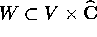which is the closure of the set of (v,z) such that. The function fieldwheredenotes the rational function obtained by projecting W to.

W may be thought of as the graph of a multi-valued function W(v) which sends v to the roots of. We call such a multi-valued map a rational correspondence.

We say W is a rigid correspondence if its set of values assumes only one conformal configuration on the Riemann sphere: i.e. there exists a finite setsuch that the set W(v) is equal tofor some Möbius transformationdepending on v. In this case we say the field extensionis a rigid extension.

Now let k' denote a finite Galois extension of k with Galois group G.

T 2.1. The field extension k'/k is the splitting field of a rigid extension if and only if there exists:

(a)
a faithful homomorphism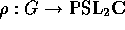and
(b)
an elementinsuch that
(c)for all g in G.

Proof. Let k' be the splitting field of a rigid correspondence. For simplicity, assumeis at least 3. Let, i = 1,2,3 denote three distinct conjugates ofunder G.acts triply transitively on the projective line; taketo be the unique group element which movesto.

We claim thatis infor all g in G. Indeed,is just the cross-ratio of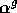and, which is constant by rigidity. Let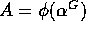be the image underof the conjugates of.

Define. Thenpermutes A, so it is an element of. Because G acts trivially on,is a homomorphism; e.g.and sincefixes A pointwise only if g fixes the conjugates of, it is faithful; thus we have verified (a-c).

Conversely, given the data (a-c), setfor any x inwith trivial stabilizer in; thenis rigid over k and.

Cohomological Interpretation. The mapdetermines an elementof the Galois cohomology group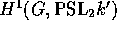, which is naturally a subgroup of the Brauer group of k; condition (c) simply saysis the coboundary of, so.

A geometric formulation of the vanishing of this class is the following. Letdenote the rational map of varieties corresponding to the field extension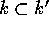. Form the Severi-Brauer variety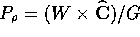, where G acts on W by birational transformations and onvia the representation. Then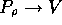is a flatbundle outside the branch locus of the map. We can factorthrough the inclusionfor any x inwith trivial stabilizer.

The cohomology class ofvanishes if and only ifis birational to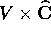; in which case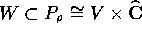presents W as a rigid correspondence.

More on Galois cohomology and interpretations of the Brauer group can be found in ,  and .Next: Purely Iterative Algorithms. Up: Solving the quintic by Previous: Introduction.

Peter Doyle